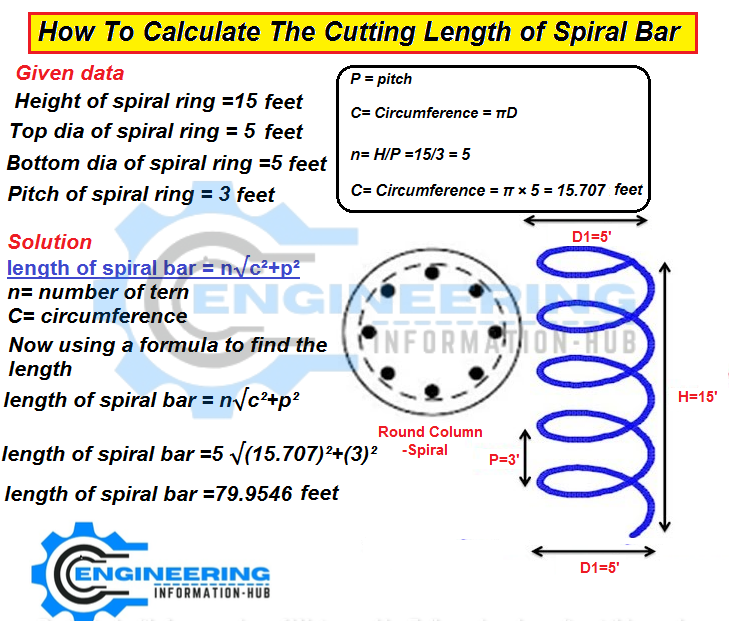# Calculate The Cutting Length of Spiral Bar |What Is The Spiral Bar And Its Uses

## Calculate The CuttingLength of Spiral Bar |What Is The SpiralBar And Its Uses

In this article, I have the topic of the spiral bar length. I will also discuss what is the spiral bar and where it uses.  Calculate The Cutting Length of the Spiral Bar |What Is The Spiral Bar And Its Uses.

### What Is The Spiral Bar And Its Uses?

Helical Spiral bars are designed for playing permanent and reliable crack sewing repairs to masonry walls and masonry. they’re put in across cracks in a very wall to extend the tensile and flexural strength of the masonry.

### How To Calculate The Cutting Length of Spiral Bar

Now We Calculate The Cutting Length of Spiral Bar### Given data

Height of spiral ring =15 foot

Top dia of spiral ring = 5 foot

Bottom dia of spiral ring =5 foot

Pitch of spiral ring = 3 foot

### Solution

The basic formula for Calculation of cutting length of the spiral bar

##### length of spiral bar = n√c²+p²

n= number of tern

C= circumference

P = pitch

C= Circumference = πD

C= Circumference of spiral bar = π × 5 = 15.707 foot

n= H/P =15/3 = 5

Now using a formula to find the length

length of spiral bar = n√c²+p²

length of spiral bar =5 √(15.707)²+(3)²

length of spiral bar =79.9546 foot

THANKS

### Raja Numan

Hi, My name is Engr. Raja Numan author of Engineering Information Hub and I am a Civil Engineer by Profession and I've specialized in the field of Quantity Surveying, Land Surveying as QC Engineer in national and multinational companies of Pakistan & Saudi Arabia.

### One Comment

1.Faizan says:

Hi dear engineering hub,
I need the method of finding Irregular shaped land and angles of that?
If you don’t mind please send on my whatsapp # 0093700340780

Check Also
Close
error: Content is protected !!